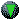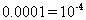Conversion Factors for Areas

 Conversion Factors   for Area  The factors are for converting to SI units (square meters). "Solar energy" is really solar power per unit area.  Various authors have used various units of area ---  everything from square centimeters to square miles.

 multiply number ofbyto get square meters square centimeterssquare feet 0.092 square yards 0.836 hectares 10,000 square miles 2,590,000 acres 4047